# Fraction calculator

The calculator performs basic and advanced operations with fractions, expressions with fractions combined with integers, decimals, and mixed numbers. It also shows detailed step-by-step information about the fraction calculation procedure. Solve problems with two, three, or more fractions and numbers in one expression.

## Result:

### 10 - 65/6 = 19/6 = 3 1/6 ≅ 3.1666667

Spelled result in words is nineteen sixths (or three and one sixth).

### How do you solve fractions step by step?

1. Conversion a mixed number 6 5/6 to a improper fraction: 6 5/6 = 6 5/6 = 6 · 6 + 5/6 = 36 + 5/6 = 41/6

To find a new numerator:
a) Multiply the whole number 6 by the denominator 6. Whole number 6 equally 6 * 6/6 = 36/6
b) Add the answer from previous step 36 to the numerator 5. New numerator is 36 + 5 = 41
c) Write a previous answer (new numerator 41) over the denominator 6.

Six and five sixths is forty-one sixths
2. Subtract: 10 - 41/6 = 10/1 - 41/6 = 10 · 6/1 · 6 - 41/6 = 60/6 - 41/6 = 60 - 41/6 = 19/6
For adding, subtracting, and comparing fractions, it is suitable to adjust both fractions to a common (equal, identical) denominator. The common denominator you can calculate as the least common multiple of both denominators - LCM(1, 6) = 6. In practice, it is enough to find the common denominator (not necessarily the lowest) by multiplying the denominators: 1 × 6 = 6. In the following intermediate step, it cannot further simplify the fraction result by canceling.
In other words - ten minus forty-one sixths = nineteen sixths.

#### Rules for expressions with fractions:

Fractions - simply use a forward slash between the numerator and denominator, i.e., for five-hundredths, enter 5/100. If you are using mixed numbers, be sure to leave a single space between the whole and fraction part.
The slash separates the numerator (number above a fraction line) and denominator (number below).

Mixed numerals (mixed fractions or mixed numbers) write as integer separated by one space and fraction i.e., 1 2/3 (having the same sign). An example of a negative mixed fraction: -5 1/2.
Because slash is both signs for fraction line and division, we recommended use colon (:) as the operator of division fractions i.e., 1/2 : 3.

Decimals (decimal numbers) enter with a decimal point . and they are automatically converted to fractions - i.e. 1.45.

The colon : and slash / is the symbol of division. Can be used to divide mixed numbers 1 2/3 : 4 3/8 or can be used for write complex fractions i.e. 1/2 : 1/3.
An asterisk * or × is the symbol for multiplication.
Plus + is addition, minus sign - is subtraction and ()[] is mathematical parentheses.
The exponentiation/power symbol is ^ - for example: (7/8-4/5)^2 = (7/8-4/5)2

#### Examples:

subtracting fractions: 2/3 - 1/2
multiplying fractions: 7/8 * 3/9
dividing Fractions: 1/2 : 3/4
exponentiation of fraction: 3/5^3
fractional exponents: 16 ^ 1/2
adding fractions and mixed numbers: 8/5 + 6 2/7
dividing integer and fraction: 5 ÷ 1/2
complex fractions: 5/8 : 2 2/3
decimal to fraction: 0.625
Fraction to Decimal: 1/4
Fraction to Percent: 1/8 %
comparing fractions: 1/4 2/3
multiplying a fraction by a whole number: 6 * 3/4
square root of a fraction: sqrt(1/16)
reducing or simplifying the fraction (simplification) - dividing the numerator and denominator of a fraction by the same non-zero number - equivalent fraction: 4/22
expression with brackets: 1/3 * (1/2 - 3 3/8)
compound fraction: 3/4 of 5/7
fractions multiple: 2/3 of 3/5
divide to find the quotient: 3/5 ÷ 2/3

The calculator follows well-known rules for order of operations. The most common mnemonics for remembering this order of operations are:
PEMDAS - Parentheses, Exponents, Multiplication, Division, Addition, Subtraction.
BEDMAS - Brackets, Exponents, Division, Multiplication, Addition, Subtraction
BODMAS - Brackets, Of or Order, Division, Multiplication, Addition, Subtraction.
GEMDAS - Grouping Symbols - brackets (){}, Exponents, Multiplication, Division, Addition, Subtraction.
Be careful, always do multiplication and division before addition and subtraction. Some operators (+ and -) and (* and /) has the same priority and then must evaluate from left to right.

## Fractions in word problems:

• Math testObelix filled a mathematical test in which he answered 25 questions. For every correct answer, he received 5 points, for each bad answer he had 3 points deducted. Obelix gained 36% of all points in the test. How many questions did he solve correctly?
• Package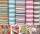The package was 23 meters of textile. The first day sold 12.3 meters. How many meters of textile remained in the package?About 6/9 of the sixth- grade pupils will be going to the parents' seminar. If 1/6 of the participants are girls, what part of the portion of sixth graders are boys?
• Of the 2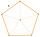Of the shapes,1/6 are triangles, and 5/12 are pentagons. What fraction of the shapes are either triangles or pentagons?
• IssacIssac eats 1/6 of the pizza. Maya then eats 3/5 of the remaining pizza. What fraction of the original pizza is left?
• Rita hasRita has 3/4 m of Ifugao cloth. She used 2/3 m for placement. What part of Ifugao cloth was left?
• CerealsAri and Joey share a 30-ounce box of cereal. By the end of the week, Ari has eaten 3/10 of the box, and Joey has eaten 3/5 of the box of cereal. How many ounces are left in the box?
• Math: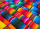Math: solve each problem. 1. for the school's intramurals, a group of students prepared 23 1/2 liters of lemonade to sell. They had 3 5/8 liters leftover. How many liters of lemonade were sold? 2. My mother bought 3 3/4 kg of beef, 23/5 kg of pork, and 5
• Bathroom 4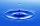Dolor puts 3 1/2 pails of water into a water container in the bathroom every day. Her daughter, Lei, uses 2 1/4 pails of water every day when taking a bath. If the water container had 5 5/8 pails of water at the start, how much water is left in it after 5
• PediatricianPediatrician this month of 20 working days takes 8 days holidays. What is the probability that on Monday it will be at work?
• The Mayflower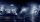The Mayflower traveled for 66 days on the trip from England to America. The weather was storming for many days of their trip. If one and a half of the days at Sea where Sunny with good weather, 1/6 of the days were sunny but very windy and the other days
• Fraction expressionWhich expression is equivalent to : minus 9 minus left parenthesis minus 4 start fraction 1 divided by 3 end fraction right parenthesis
• Product and sumWhat is the product of two fourths  and the sum of three halves and four?